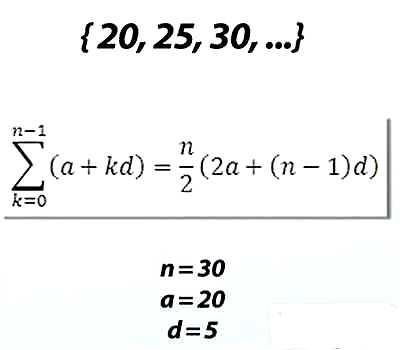Selection of Terms in an Arithmetic Progression

A sequence of numbers is known as an arithmetic progression (A.P.) if the difference between the term and the preceding term is always same or constant.

An arithmetic progression is a sequence of numbers such that the difference between any two successive members is constant.

For example,

• the sequence 1, 2, 3, 4, … is an arithmetic progression with common difference 1.
• the sequence 3, 5, 7, 9, 11,… is an arithmetic progression with common difference 2.
• the sequence 20, 10, 0, -10, -20, -30, … is an arithmetic progression with common difference -10.Sometimes we need to assume a certain number of terms in Arithmetic Progression. The following ways are generally used for the selection of terms in an arithmetic progression.

(i) If the sum of three terms in Arithmetic Progression is given, assume the numbers as a – d, a and a + d. Here the common difference is d.

(ii) If the sum of four terms in Arithmetic Progression is given, assume the numbers as a – 3d, a – d, a + d and a + 3d.

(iii) If the sum of five terms in Arithmetic Progression is given, assume the numbers as a – 2d, a – d, a, a + d and a + 2d. Here the common difference is 2d.

(iv) If the sum of six terms in Arithmetic Progression is given, assume the numbers as a – 5d, a – 3d, a – d, a + d, a + 3d and a + 5d. Here the common difference is 2d.

Note: From the above explanation we understand that in case of an odd number of terms, the middle term is ‘a’ and the common difference is ‘d’.

Again, in case of an even number of terms the middle terms are a – d, a + d and the common difference is 2d.

If you have the sequence 2, 8, 14, 20, 26, then each term is 6 more than the previous term. This is an example of an arithmetic progression (AP) and the constant value that defines the difference between any two consecutive terms is called the common difference.

If an arithmetic difference has a first term ‘a’ and a common difference of d, then we can write

a, (a + d), (a + 2d),… {a + (n-1) d}

where the nth  term = a + (n−1)d.

‘a’ is the first term, and

‘d’ is the difference between the terms (called the “common difference”)

Information Source: